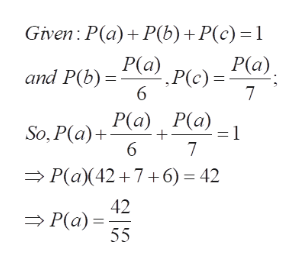# If S = {a, b, c} with P(a) = 6P(b) = 7P(c), find P(a).

Question
9 views

If S = {abc} with P(a) = 6P(b) = 7P(c), find P(a).

check_circle

Step 1

To work out the required probability , using the basic equations

Step 2

Key point: there are three events a, b and c, and their combined pro...help_outlineImage TranscriptioncloseGiven: P(a) P(b)+P(c) = 1 and P(b) a) Pc)= P(a) 6 7 So, P(a)+a) P(a) 6 7 P(a)(42 7+6)= 42 42 P(a) 55 fullscreen

### Want to see the full answer?

See Solution

#### Want to see this answer and more?

Solutions are written by subject experts who are available 24/7. Questions are typically answered within 1 hour.*

See Solution
*Response times may vary by subject and question.
Tagged in

### Basic Probability